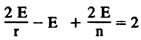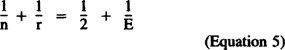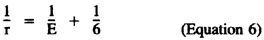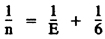﻿ The Five Pythagorean Solids - Cosmos - Carl Sagan ﻿

## Cosmos - Carl Sagan (1980)

### APPENDIX 2. The Five Pythagorean Solids

A regular polygon (Greek for “many-angled”) is a two-dimensional figure with some number, n, of equal sides. So n = 3 is an equilateral triangle, n = 4 is a square, n = 5 is a pentagon, and so on. A polyhedron (Greek for “many-sided”) is a three-dimensional figure, all of whose faces are polygons: a cube, for example, with 6 squares for faces. A simple polyhedron, or regular solid, is one with no holes in it. Fundamental to the work of the Pythagoreans and of Johannes Kepler was the fact that there can be 5 and only 5 regular solids. The easiest proof comes from a relationship discovered much later by Descartes and by Leonhard Euler which relates the number of faces, F, the number of edges, E, and the number of corners or vertices, V, of a regular solid:

V – E + F = 2     (Equation 2)

So for a cube, there are 6 faces (F = 6) and 8 vertices (V = 8), and 8 – E + 6 = 2, 14 – E = 2, and E = 12; Equation (2) predicts that the cube has 12 edges, as it does. A simple geometric proof of Equation (2) can be found in the book by Courant and Robbins in the Bibliography. From Equation (2) we can prove that there are only five regular solids:

Every edge of a regular solid is shared by the sides of two adjacent polygons. Think again of the cube, where every edge is a boundary between two squares. If we count up all the sides of all the faces of a polyhedron, n F, we will have counted every edge twice. So

n F = 2 E     (Equation 3)

Let r represent how many edges meet at each vertex. For a cube, r = 3. Also, every edge connects two vertices. If we count up all the vertices, r V, we will similarly have counted every edge twice. So

r V = 2 E     (Equation 4)

Substituting for V and F in Equation (2) from Equations (3) and (4), we findIf we divide both sides of this equation by 2 E, we haveWe know that n is 3 or more, since the simplest polygon is the triangle, with three sides. We also know that r is 3 or more, since at least 3 faces meet at a given vertex in a polyhedron. If both n and r were simultaneously more than 3, the left-hand side of Equation (5) would be less than ⅔ and the equation could not be satisfied for any positive value of E. Thus, by another reductio ad absurdum argument, either n = 3 and r is 3 or more, or r = 3 and n is 3 or more.

If n = 3, Equation (5) becomes (1/3) + (1/r) = (1/2) + (1/E), orSo in this case r can equal 3, 4, or 5 only. (If E were 6 or more, the equation would be violated.) Now n = 3, r = 3 designates a solid in which 3 triangles meet at each vertex. By Equation (6) it has 6 edges; by Equation (3) it has 4 faces; by Equation (4) it has 4 vertices. Clearly it is the pyramid or tetrahedron; n = 3, r = 4 is a solid with 8 faces in which 4 triangles meet at each vertex, the octahedron; and n = 3, r = 5 represents a solid with 20 faces in which 5 triangles meet at each vertex, the icosahedron (see figures on this page).

If r = 3, Equation (5) becomesand by similar arguments n can equal 3, 4, or 5 only, n = 3 is the tetrahedron again; n = 4 is a solid whose faces are 6 squares, the cube; and n = 5 corresponds to a solid whose faces are 12 pentagons, the dodecahedron.

There are no other integer values of n and r possible, and therefore there are only 5 regular solids, a conclusion from abstract and beautiful mathematics that has had, as we have seen, the most profound impact on practical human affairs.

﻿

﻿